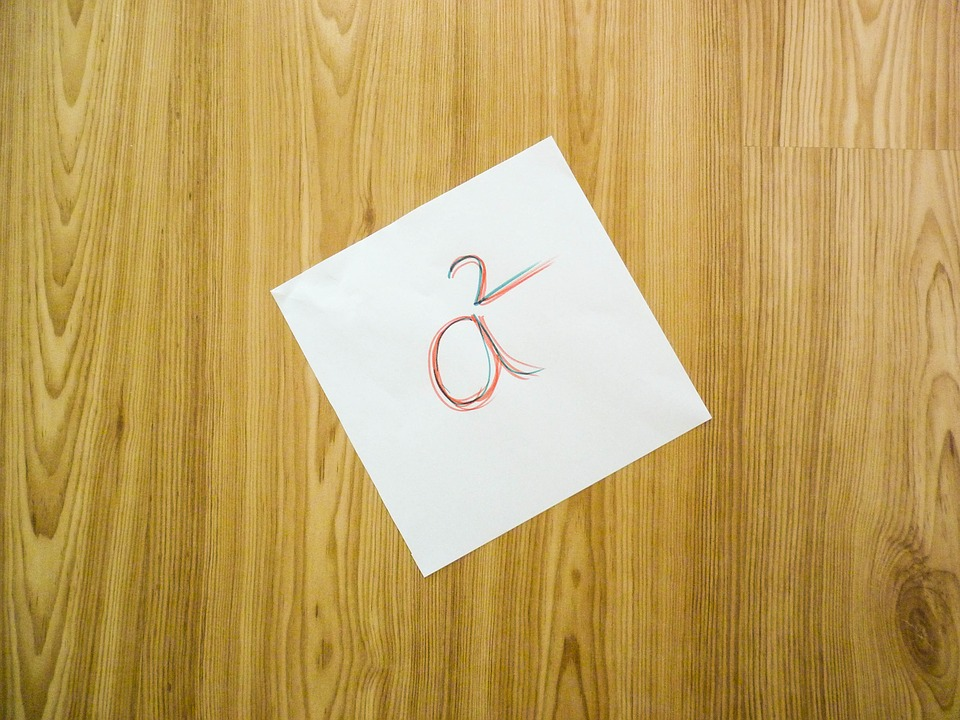# Perfect the Square Method Class 10th

## Perfect The Square Method (Complete The Square Method)

### Introduction

In the Perfect the Square Method, we convert the quadratic equation in the perfect square form and then we take the square root to obtain the required roots.

This method will be clear by the following examples.

#### Examples

Example – 1) Solve the quadratic equation 9x2 – 3x – 2 = 0 by the perfect square method.

Solution – Given equation 9x2 – 3x – 2 = 0

Step 1) We divide the whole equation by 9 to make 1 as the coefficient of x2.

9x2/9 – 3x/9 – 2/9 = 0

x2 – x/3 – 2/9 = 0

Step 2) Taking the constant term to the right side

x2 – x/3 = 2/9

Step 3) Adding the square of half of the coefficient of x to both sides to make a perfect square on the left side.

x2 – x/3 + (⅙)2 = 2/9 + (⅙)2             [Half of coefficient of x = ⅓ ÷ 2 = ⅓⨯½ = ⅙]

Step 4) Now the left side is a perfect square.

Easy Tip – To make a perfect square, we put the sign of the middle term (– x/3) of the equation (x2 – x/3 – 2/9 = 0) which in this example is – (negative), between the variable x and half of the coefficient of x which is ⅙, and make a perfect square (x – ⅙)2.

(x – ⅙)2 = 2/9 + 1/36

(x – ⅙)2 = (2⨯4 + 1⨯1)/36 (LCM = 36)

(x – ⅙)2 = 9/36

(x – ⅙)2 = ¼

Step 5) Taking square root of both sides.

√(x – ⅙)2 = √¼

x – ⅙ = ±½

Taking both the values separately,

On taking the (+) sign,

x – ⅙ = +½

x = ½ + ⅙

x = (1⨯3 + 1⨯1)/6

x = 4/6

x = ⅔

On taking the (−) sign,

x – ⅙ = −½

x = −½ + ⅙

x = (−1⨯3 + 1⨯1)/6

x = −2/6

x = −⅓

So, x = ⅔ and x = −⅓ are the required roots of the quadratic equation 9x2 – 3x – 2 = 0.    Ans.

Example – 2) Solve the quadratic equation 2x2 – 7x + 3 = 0 by the perfect square method.

Solution – Given equation 2x2 – 7x + 3 = 0

Dividing the equation by 2 to make 1 as the coefficient of x2

2x2/2 – 7x/2 + 3/2 = 0

x2 – 7x/2 + 3/2 = 0

Taking the constant term to right side

x2 – 7x/2 = –3/2

Adding the square of half of the coefficient of x to both sides

x2 – 7x/2 + (7/4)2 = –3/2 + (7/4)2 [Half of coefficient of x = 7/2 ÷ 2 = 7/2⨯½ = 7/4]

(x – 7/4)2 = –3/2 + 49/16 = (–3⨯8 + 49⨯1)/16 = (–24 + 49)/16 = 25/16

(x – 7/4)2 = 25/16

Taking square root both sides,

√(x – 7/4)2 = √(25/16)

x – 7/4 = ±5/4

On taking the (+) sign,

x – 7/4 = +5/4

x = 5/4 + 7/4

x = (5 + 7)/4 = 12/4

x = 3

On taking the (–) sign,

x – 7/4 = –5/4

x = –5/4 + 7/4

x = (–5 + 7)/4 = 2/4

x = ½

Both the values of the x are the required roots.  Ans.

Perfect the Square Method Class 10th in Hindi

More About Perfect the Square Method

Rate this post